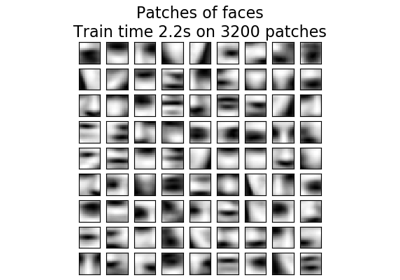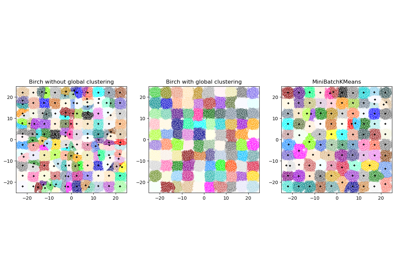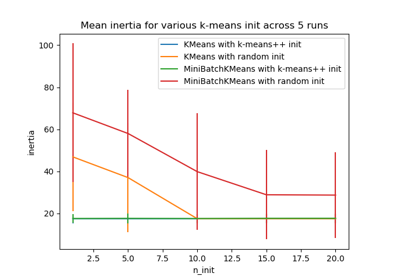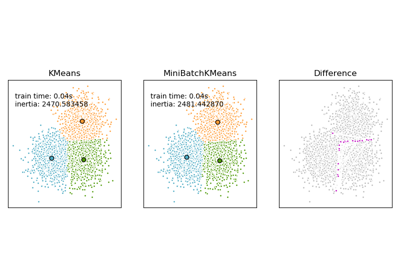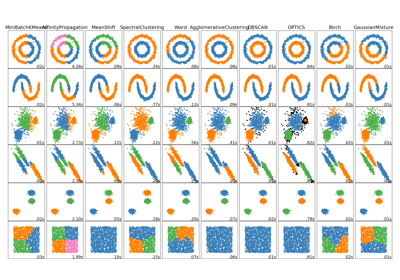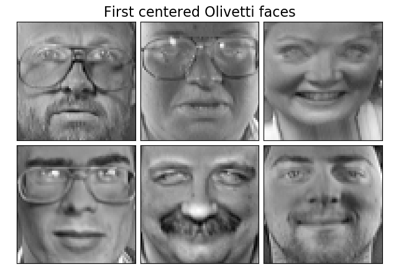# sklearn.cluster.MiniBatchKMeans¶

class sklearn.cluster.MiniBatchKMeans(n_clusters=8, init='k-means++', max_iter=100, batch_size=100, verbose=0, compute_labels=True, random_state=None, tol=0.0, max_no_improvement=10, init_size=None, n_init=3, reassignment_ratio=0.01)[source]

Mini-Batch K-Means clustering.

Read more in the User Guide.

Parameters
n_clustersint, default=8

The number of clusters to form as well as the number of centroids to generate.

init{‘k-means++’, ‘random’} or ndarray of shape (n_clusters, n_features), default=’k-means++’

Method for initialization

‘k-means++’ : selects initial cluster centers for k-mean clustering in a smart way to speed up convergence. See section Notes in k_init for more details.

‘random’: choose k observations (rows) at random from data for the initial centroids.

If an ndarray is passed, it should be of shape (n_clusters, n_features) and gives the initial centers.

max_iterint, default=100

Maximum number of iterations over the complete dataset before stopping independently of any early stopping criterion heuristics.

batch_sizeint, default=100

Size of the mini batches.

verboseint, default=0

Verbosity mode.

compute_labelsbool, default=True

Compute label assignment and inertia for the complete dataset once the minibatch optimization has converged in fit.

random_stateint, RandomState instance, default=None

Determines random number generation for centroid initialization and random reassignment. Use an int to make the randomness deterministic. See Glossary.

tolfloat, default=0.0

Control early stopping based on the relative center changes as measured by a smoothed, variance-normalized of the mean center squared position changes. This early stopping heuristics is closer to the one used for the batch variant of the algorithms but induces a slight computational and memory overhead over the inertia heuristic.

To disable convergence detection based on normalized center change, set tol to 0.0 (default).

max_no_improvementint, default=10

Control early stopping based on the consecutive number of mini batches that does not yield an improvement on the smoothed inertia.

To disable convergence detection based on inertia, set max_no_improvement to None.

init_sizeint, default=None

Number of samples to randomly sample for speeding up the initialization (sometimes at the expense of accuracy): the only algorithm is initialized by running a batch KMeans on a random subset of the data. This needs to be larger than n_clusters.

If None, init_size= 3 * batch_size.

n_initint, default=3

Number of random initializations that are tried. In contrast to KMeans, the algorithm is only run once, using the best of the n_init initializations as measured by inertia.

reassignment_ratiofloat, default=0.01

Control the fraction of the maximum number of counts for a center to be reassigned. A higher value means that low count centers are more easily reassigned, which means that the model will take longer to converge, but should converge in a better clustering.

Attributes
cluster_centers_ndarray of shape (n_clusters, n_features)

Coordinates of cluster centers

labels_int

Labels of each point (if compute_labels is set to True).

inertia_float

The value of the inertia criterion associated with the chosen partition (if compute_labels is set to True). The inertia is defined as the sum of square distances of samples to their nearest neighbor.

KMeans

The classic implementation of the clustering method based on the Lloyd’s algorithm. It consumes the whole set of input data at each iteration.

Notes

Examples

>>> from sklearn.cluster import MiniBatchKMeans
>>> import numpy as np
>>> X = np.array([[1, 2], [1, 4], [1, 0],
...               [4, 2], [4, 0], [4, 4],
...               [4, 5], [0, 1], [2, 2],
...               [3, 2], [5, 5], [1, -1]])
>>> # manually fit on batches
>>> kmeans = MiniBatchKMeans(n_clusters=2,
...                          random_state=0,
...                          batch_size=6)
>>> kmeans = kmeans.partial_fit(X[0:6,:])
>>> kmeans = kmeans.partial_fit(X[6:12,:])
>>> kmeans.cluster_centers_
array([[2. , 1. ],
[3.5, 4.5]])
>>> kmeans.predict([[0, 0], [4, 4]])
array([0, 1], dtype=int32)
>>> # fit on the whole data
>>> kmeans = MiniBatchKMeans(n_clusters=2,
...                          random_state=0,
...                          batch_size=6,
...                          max_iter=10).fit(X)
>>> kmeans.cluster_centers_
array([[3.95918367, 2.40816327],
[1.12195122, 1.3902439 ]])
>>> kmeans.predict([[0, 0], [4, 4]])
array([1, 0], dtype=int32)


Methods

 fit(self, X[, y, sample_weight]) Compute the centroids on X by chunking it into mini-batches. fit_predict(self, X[, y, sample_weight]) Compute cluster centers and predict cluster index for each sample. fit_transform(self, X[, y, sample_weight]) Compute clustering and transform X to cluster-distance space. get_params(self[, deep]) Get parameters for this estimator. partial_fit(self, X[, y, sample_weight]) Update k means estimate on a single mini-batch X. predict(self, X[, sample_weight]) Predict the closest cluster each sample in X belongs to. score(self, X[, y, sample_weight]) Opposite of the value of X on the K-means objective. set_params(self, \*\*params) Set the parameters of this estimator. transform(self, X) Transform X to a cluster-distance space.
__init__(self, n_clusters=8, init='k-means++', max_iter=100, batch_size=100, verbose=0, compute_labels=True, random_state=None, tol=0.0, max_no_improvement=10, init_size=None, n_init=3, reassignment_ratio=0.01)[source]

Initialize self. See help(type(self)) for accurate signature.

fit(self, X, y=None, sample_weight=None)[source]

Compute the centroids on X by chunking it into mini-batches.

Parameters
Xarray-like or sparse matrix, shape=(n_samples, n_features)

Training instances to cluster. It must be noted that the data will be converted to C ordering, which will cause a memory copy if the given data is not C-contiguous.

yIgnored

Not used, present here for API consistency by convention.

sample_weightarray-like, shape (n_samples,), optional

The weights for each observation in X. If None, all observations are assigned equal weight (default: None).

Returns
self
fit_predict(self, X, y=None, sample_weight=None)[source]

Compute cluster centers and predict cluster index for each sample.

Convenience method; equivalent to calling fit(X) followed by predict(X).

Parameters
X{array-like, sparse matrix} of shape (n_samples, n_features)

New data to transform.

yIgnored

Not used, present here for API consistency by convention.

sample_weightarray-like, shape (n_samples,), optional

The weights for each observation in X. If None, all observations are assigned equal weight (default: None).

Returns
labelsarray, shape [n_samples,]

Index of the cluster each sample belongs to.

fit_transform(self, X, y=None, sample_weight=None)[source]

Compute clustering and transform X to cluster-distance space.

Equivalent to fit(X).transform(X), but more efficiently implemented.

Parameters
X{array-like, sparse matrix} of shape (n_samples, n_features)

New data to transform.

yIgnored

Not used, present here for API consistency by convention.

sample_weightarray-like, shape (n_samples,), optional

The weights for each observation in X. If None, all observations are assigned equal weight (default: None).

Returns
X_newarray, shape [n_samples, k]

X transformed in the new space.

get_params(self, deep=True)[source]

Get parameters for this estimator.

Parameters
deepbool, default=True

If True, will return the parameters for this estimator and contained subobjects that are estimators.

Returns
paramsmapping of string to any

Parameter names mapped to their values.

partial_fit(self, X, y=None, sample_weight=None)[source]

Update k means estimate on a single mini-batch X.

Parameters
Xarray-like of shape (n_samples, n_features)

Coordinates of the data points to cluster. It must be noted that X will be copied if it is not C-contiguous.

yIgnored

Not used, present here for API consistency by convention.

sample_weightarray-like, shape (n_samples,), optional

The weights for each observation in X. If None, all observations are assigned equal weight (default: None).

Returns
self
predict(self, X, sample_weight=None)[source]

Predict the closest cluster each sample in X belongs to.

In the vector quantization literature, cluster_centers_ is called the code book and each value returned by predict is the index of the closest code in the code book.

Parameters
X{array-like, sparse matrix} of shape (n_samples, n_features)

New data to predict.

sample_weightarray-like, shape (n_samples,), optional

The weights for each observation in X. If None, all observations are assigned equal weight (default: None).

Returns
labelsarray, shape [n_samples,]

Index of the cluster each sample belongs to.

score(self, X, y=None, sample_weight=None)[source]

Opposite of the value of X on the K-means objective.

Parameters
X{array-like, sparse matrix} of shape (n_samples, n_features)

New data.

yIgnored

Not used, present here for API consistency by convention.

sample_weightarray-like, shape (n_samples,), optional

The weights for each observation in X. If None, all observations are assigned equal weight (default: None).

Returns
scorefloat

Opposite of the value of X on the K-means objective.

set_params(self, **params)[source]

Set the parameters of this estimator.

The method works on simple estimators as well as on nested objects (such as pipelines). The latter have parameters of the form <component>__<parameter> so that it’s possible to update each component of a nested object.

Parameters
**paramsdict

Estimator parameters.

Returns
selfobject

Estimator instance.

transform(self, X)[source]

Transform X to a cluster-distance space.

In the new space, each dimension is the distance to the cluster centers. Note that even if X is sparse, the array returned by transform will typically be dense.

Parameters
X{array-like, sparse matrix} of shape (n_samples, n_features)

New data to transform.

Returns
X_newarray, shape [n_samples, k]

X transformed in the new space.

## Examples using sklearn.cluster.MiniBatchKMeans¶